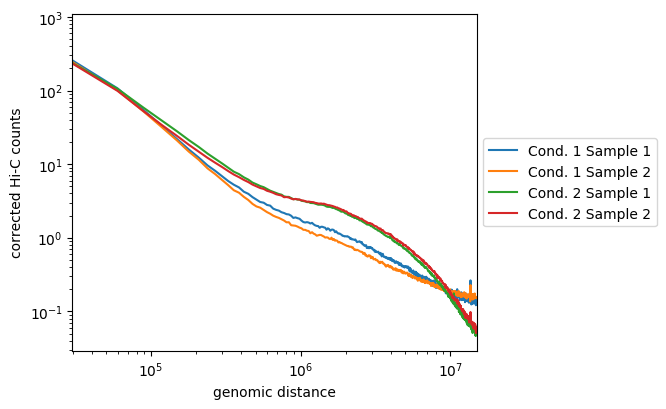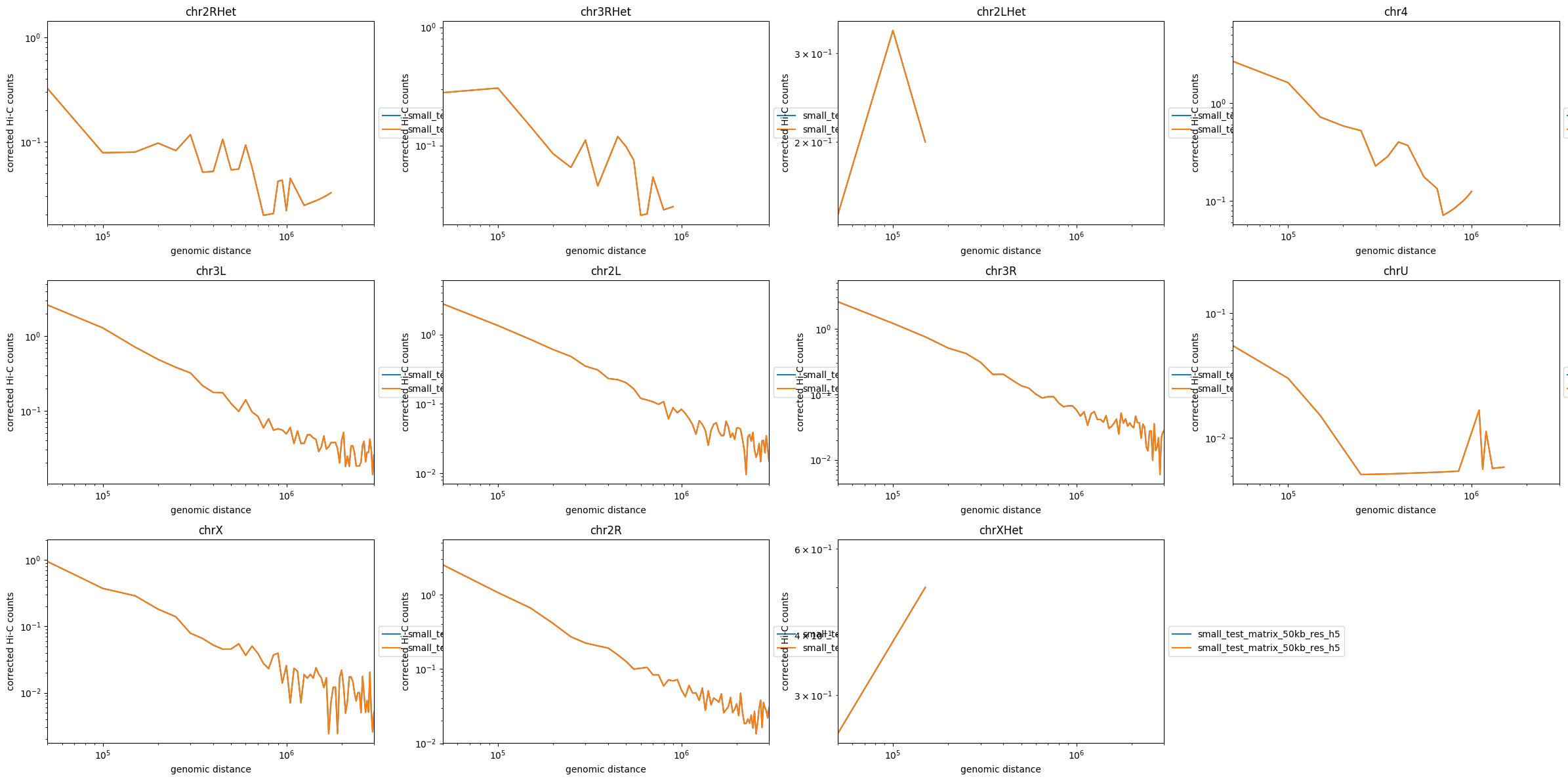Galaxy | Tool Preview

hicPlotDistVsCounts (version 3.6+galaxy1)
If set, diagonal counts are not included
If more than one Hi-C matrix is given for each chromosome a new plot is made. Otherewise, a single plot with one line per chromosome is created.
Maximum distance from diagonal to use. In other words, distances up to maxDeph are computed.
Width and height of the plot (in inches). Default is 6 * number of cols, 4 * number of rows. The maximum number of rows is 4. Example: 6 5.
Chromosomes to excludes
Chromosomes to exclude 0
A bam file containing all data underlying the plots is saved on this file.

# Relation of genomic distance and number of contacts

hicPlotDistVsCounts allows a quick comparison between multiple Hi-C matrices of the Hi-C counts enrichment at different genomic ranges / distances up to whole chromosome. Biological replicates should display the exact same distribution while samples coming from different cell-lines, treated versus untreated samples or mutant versus wild-type samples should display a different distribution at long and/or close range.

The results of this tool usually reflect the proportion of long-range and short-range contacts calculated in each sample by hicQC (which is part of hicBuildMatrix). Local TAD or contact enrichments will not impact the results computed by this tool, hicPCA is better suited for that purpose.

When plotting multiple matrices, the denser ones (more coverage) are scaled down to match the sum of the smaller matrix of the comparison.

## Usage

hicPlotDistVsCounts should be used on corrected matrices with large bins (e.g. at least 30 to 50kb bins), otherwise the curves will be spiky at longer ranges because of the sparness of the contacts, thus the likelyness of the samples will become hard to assess after a certain distance. hicPlotDistVsCounts is thus often ran after hicMergeMatrixBins and hicCorrectMatrix.

## Output

This program makes distance vs. Hi-C counts plots. It can use several matrix files to compare them. If the --perchr option is given, each chromosome is plotted independently. Below can be found an example output:Here, we see that the samples from the first condition are not so well correlated, but they follow the same tendancies and are distinct from the two samples of the second condition. The later are well correlated and display enriched long-range contacts compared to the first condition samples.

On the second graph below, the distance vs. Hi-C contact counts is computed and plotted per chromosome: